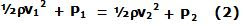Sunday, May 3, 2009

Irodov Problem 1.315At any point in the bend, as the water travels through the bend of the tube it experiences a change in the direction of its velocity vector by 90 degrees as shown in the figure from v to v'. This means that the acceleration vector of the water in this bend region dv/dt is oriented along the direction from point 1 to 2 as shown in the figure.

We know from the Eulerian equation of hydrodynamics that,Here, P is the fluid pressure,is the density of the fluid and f is the external force per unit volume acting on the fluid. Since the tube is horizontal, there is no force of gravity acting on the fluid, nor there is any other external force mentioned and hence f=0. Hence, we have,This means that the direction of increase of pressure P (gradient of P) will be in the opposite direction to that of dv/dt. Since dv/dt is directed from 1 to 2, the pressure must increase at all points as we move from 2 towards 1. This in turn means that P1 > P2.

To compare velocities at points 1 and 2 let us consider the Bernoulli's equation. We know that,Since the tube is horizontal, h1 = h2. Thus, (1) becomes,Since P1 > P2 then from equation (2) it is clear that.